[[d.project_title]][[d.material_name]]# 恩尼格玛密码机### 凡事都有个动机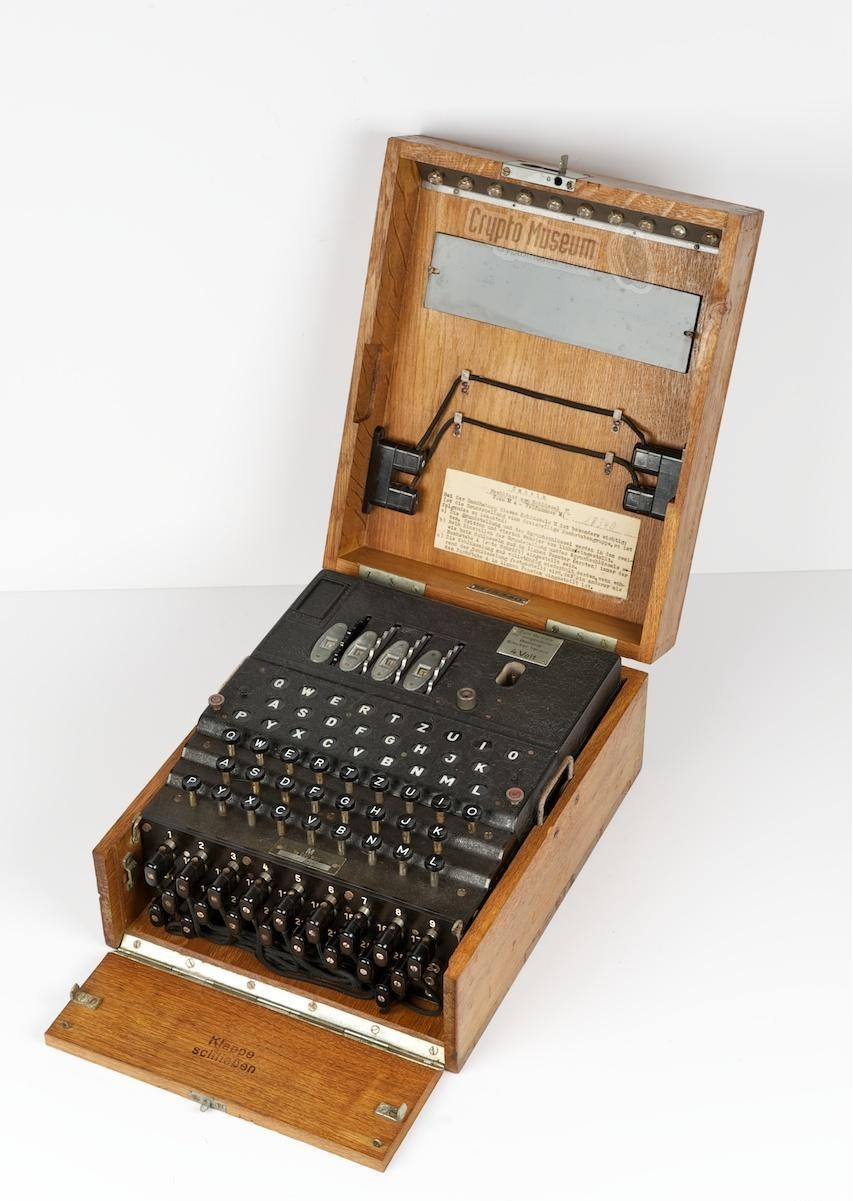### 原理

·我们把加密过程看成一条算式，有点长。

Cn=rp(r1(r2(r3(RF(R3(R2(R1((RP(Pn))))))))))    大写函数为正向，小写为逆向。RP是替换，R是转子，RF是反射器，C是密文，P是明文。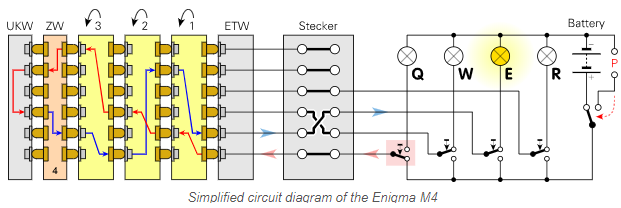### 复刻方向

#### 3.纯迷你小型化电子化

①加密解密时候密文或明文是用小灯泡显示的，在不加电传打字机的条件下并没有储存功能。

②电源采用4V直流电，可能是方便铅酸电池吧。而现在多数人使用的是5V、9V、12V。

③ 当时德国版本有29个字母按键，ASCII编码表当时完全没有影子。

④没有二极管，更没有三极管和芯片，连PCB电路板在当时也只是美军的专利。

⑤密码机复杂的程度看配套的转子数而定。量产下来一套就备有5个转子，然后跳出3个或4个使用。多几个转子在当时是要增加生产成本和复杂程度的。

#### 个人选择可以采用的电路工艺

1x
Arduino Nano
6x
74HC4067N
26x
10mm LED 白发红
27x
PBS-305复位按钮
33x
10K电阻
26x
1K电阻
26x
1N5819
26x
1N4730A
1x

1x
TP4067模块
60x
DC005插头5.5-2.1mm
52x
DC-022插座5.5-2.1mm
1x
DC-002插座
1x
USB-DC002电源线
1x
TF卡模块
1x
128MB TF卡
1x
1.3" OLED
2x
15P单排母
1x
4P单排母
1x
6P单排母
2x
6P单排针
1x
2*40P双排弯针
4x
2*3P双排母
2x
2*7P双排母
2x
2*6P双排母
6x
DIP-24插座
2x
SMTS-102C3
1x

1x
PJ-313
6x
104瓷片电容
1x
470uF电解电容
6x

1x
PCB电路板（嘉立创打板）
1x
3mm厚木板（用于激光切割外壳）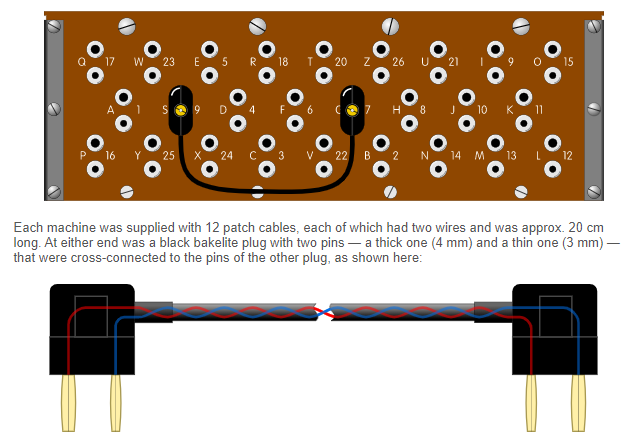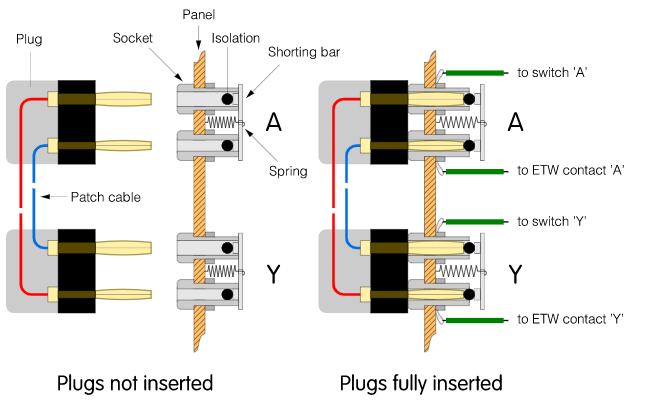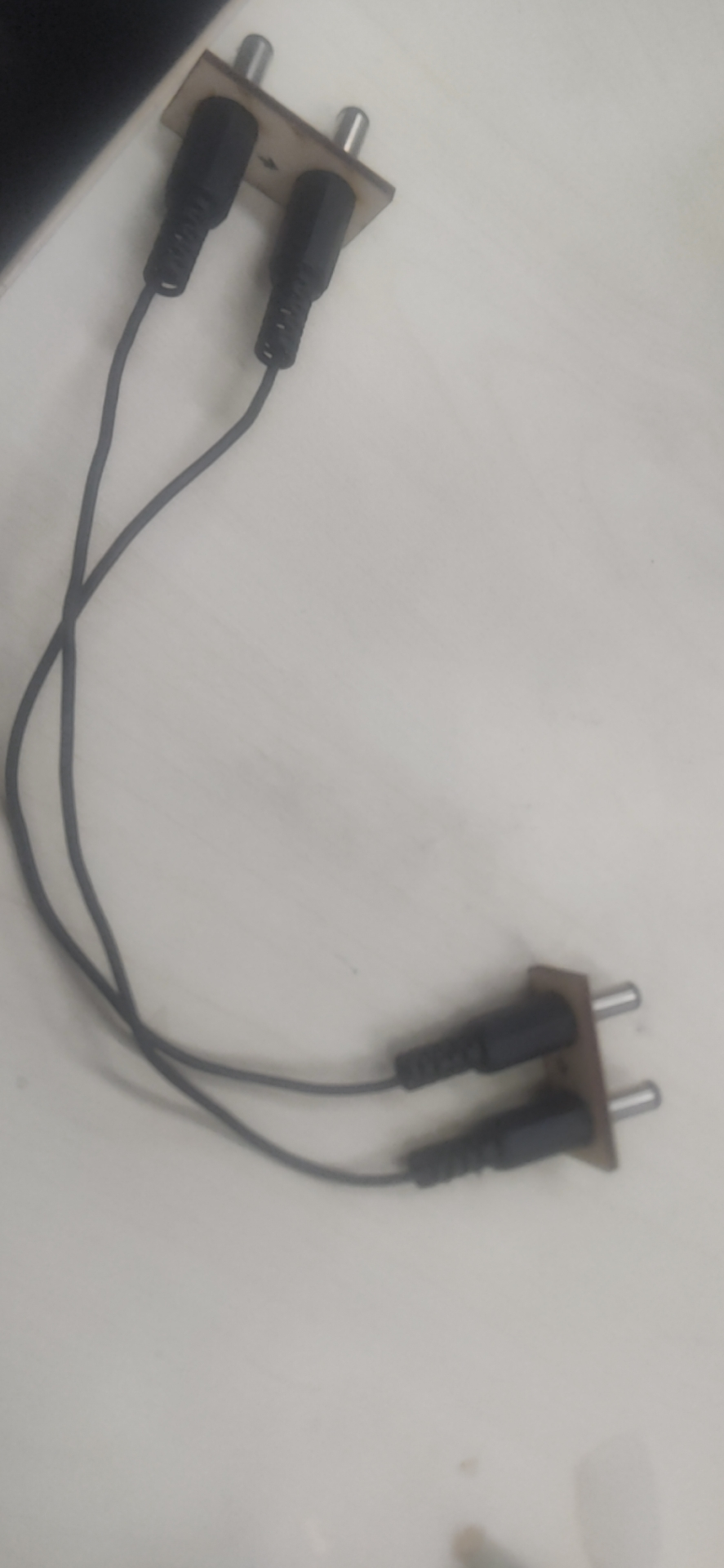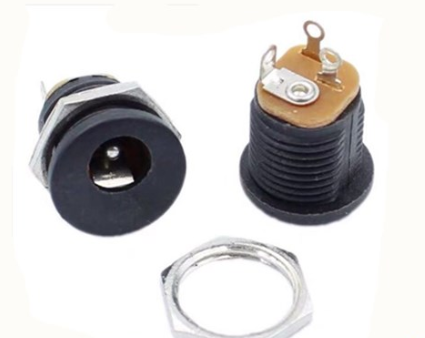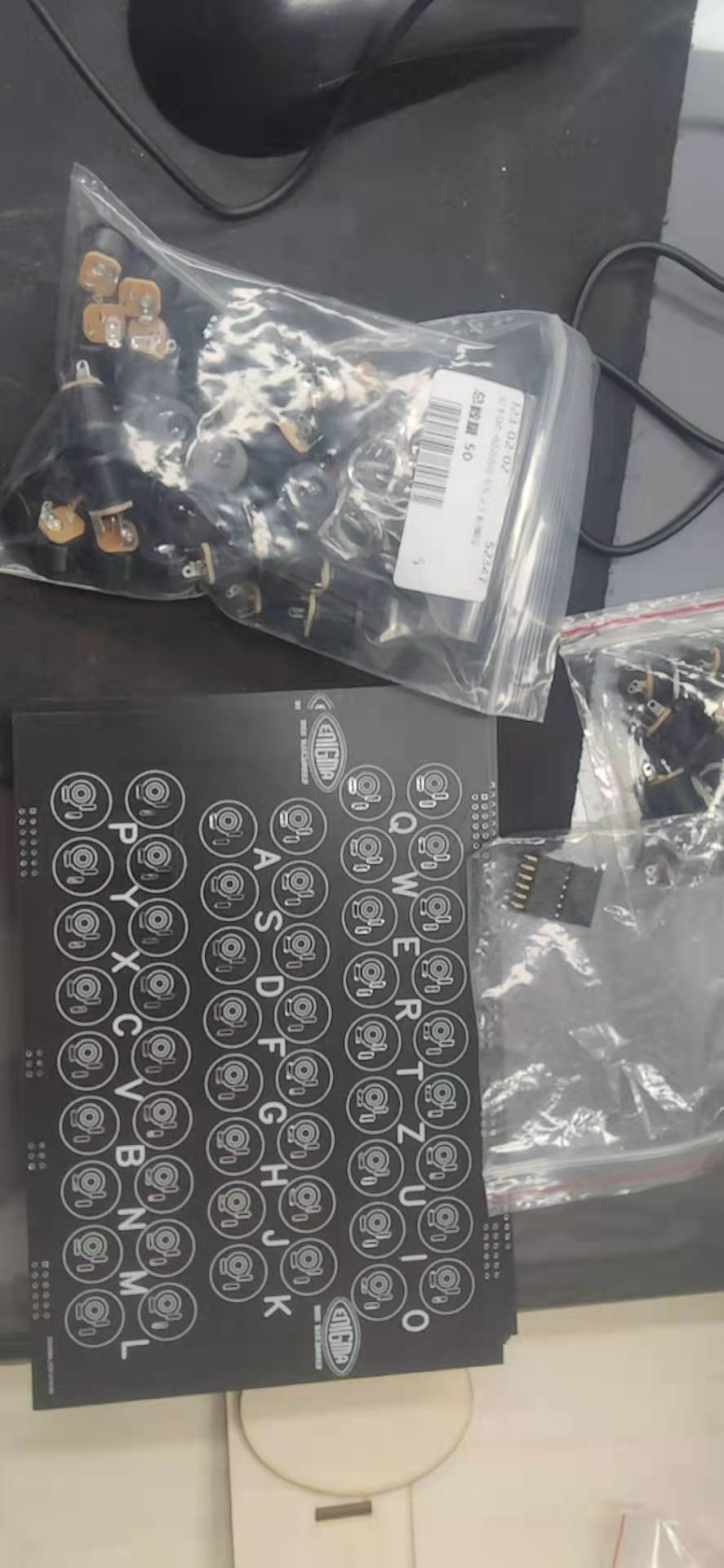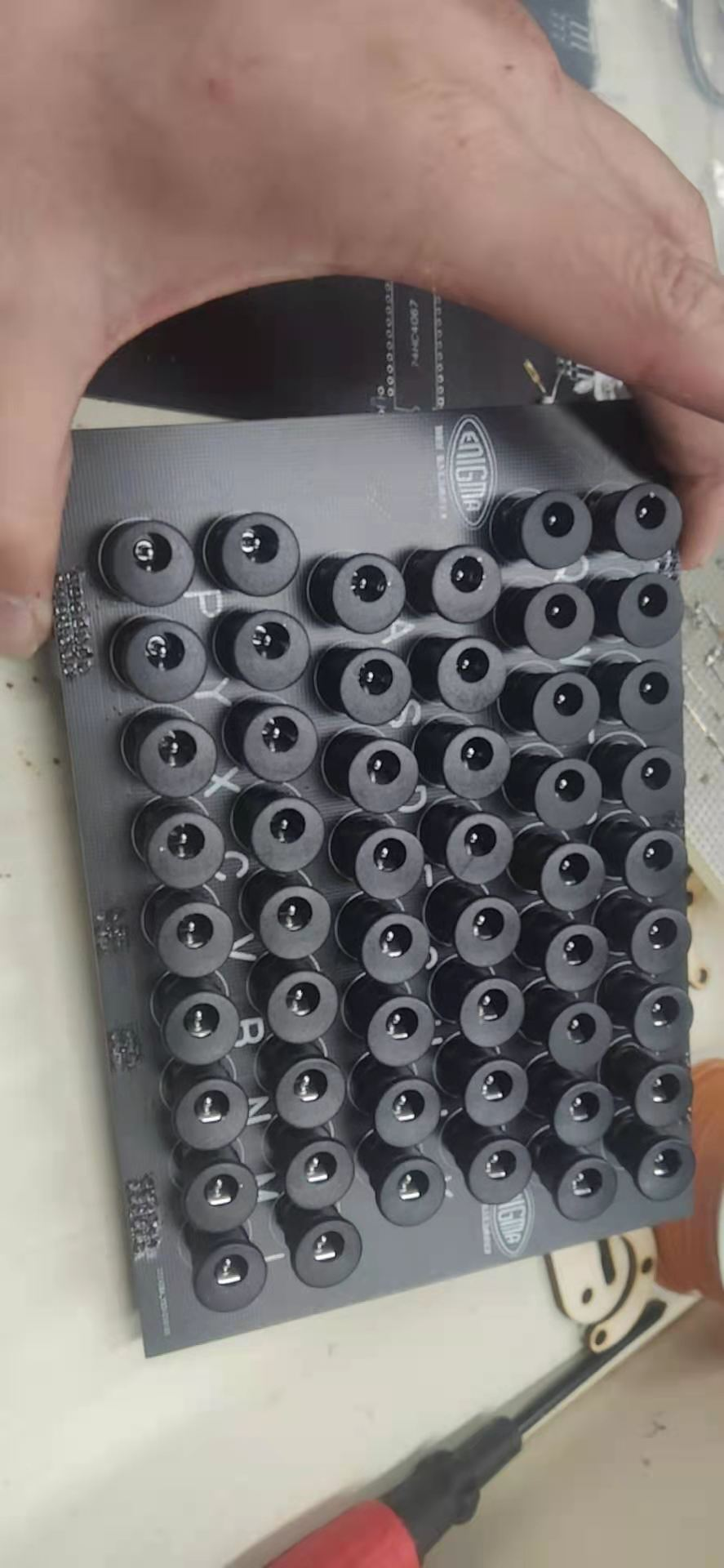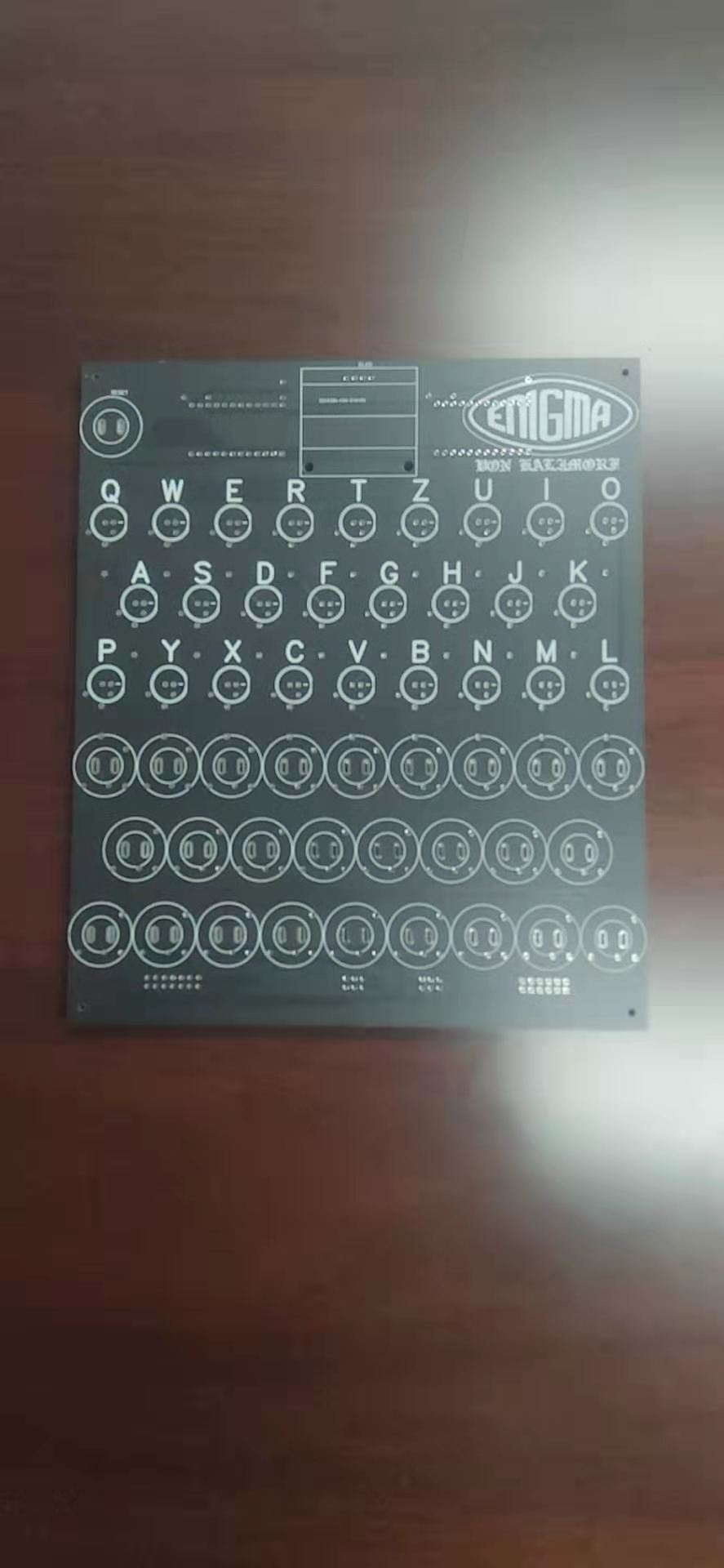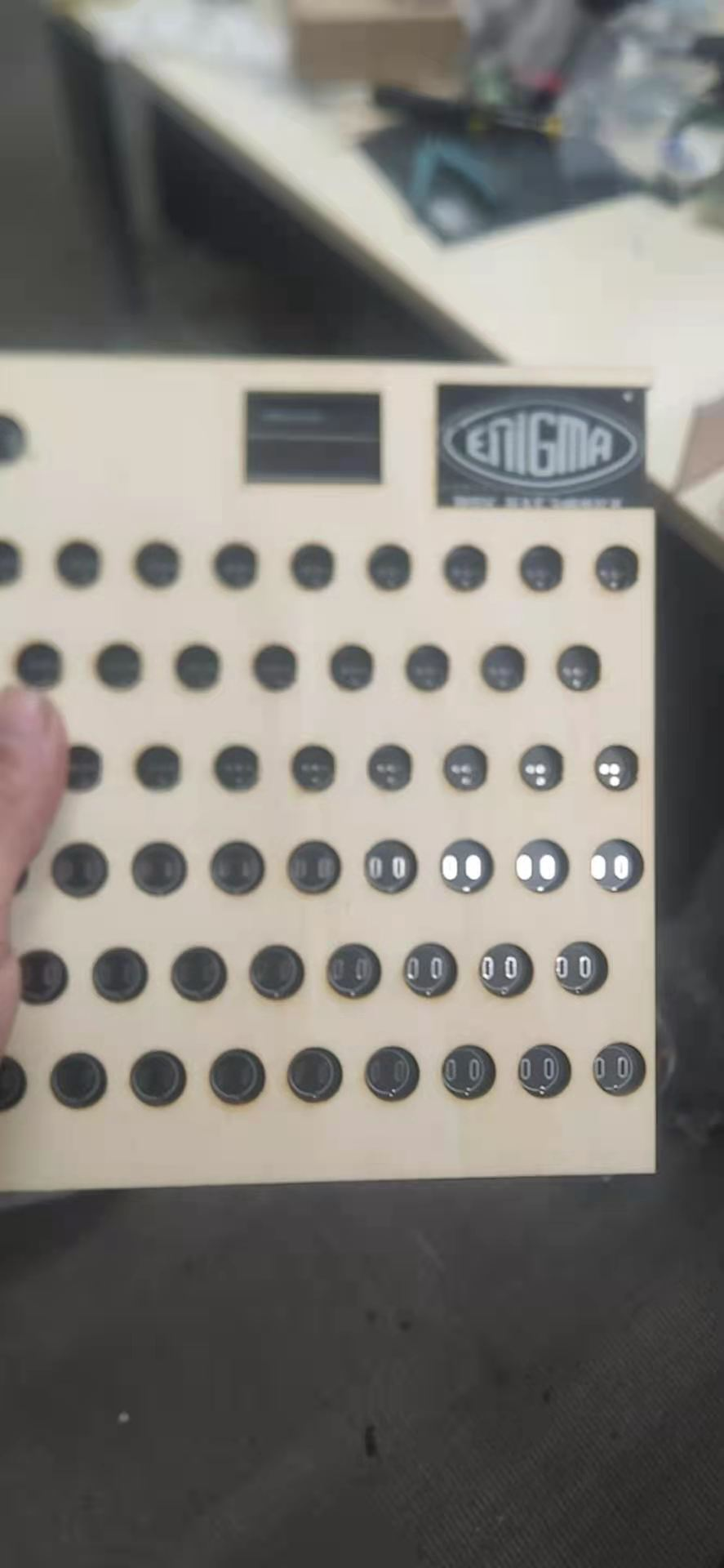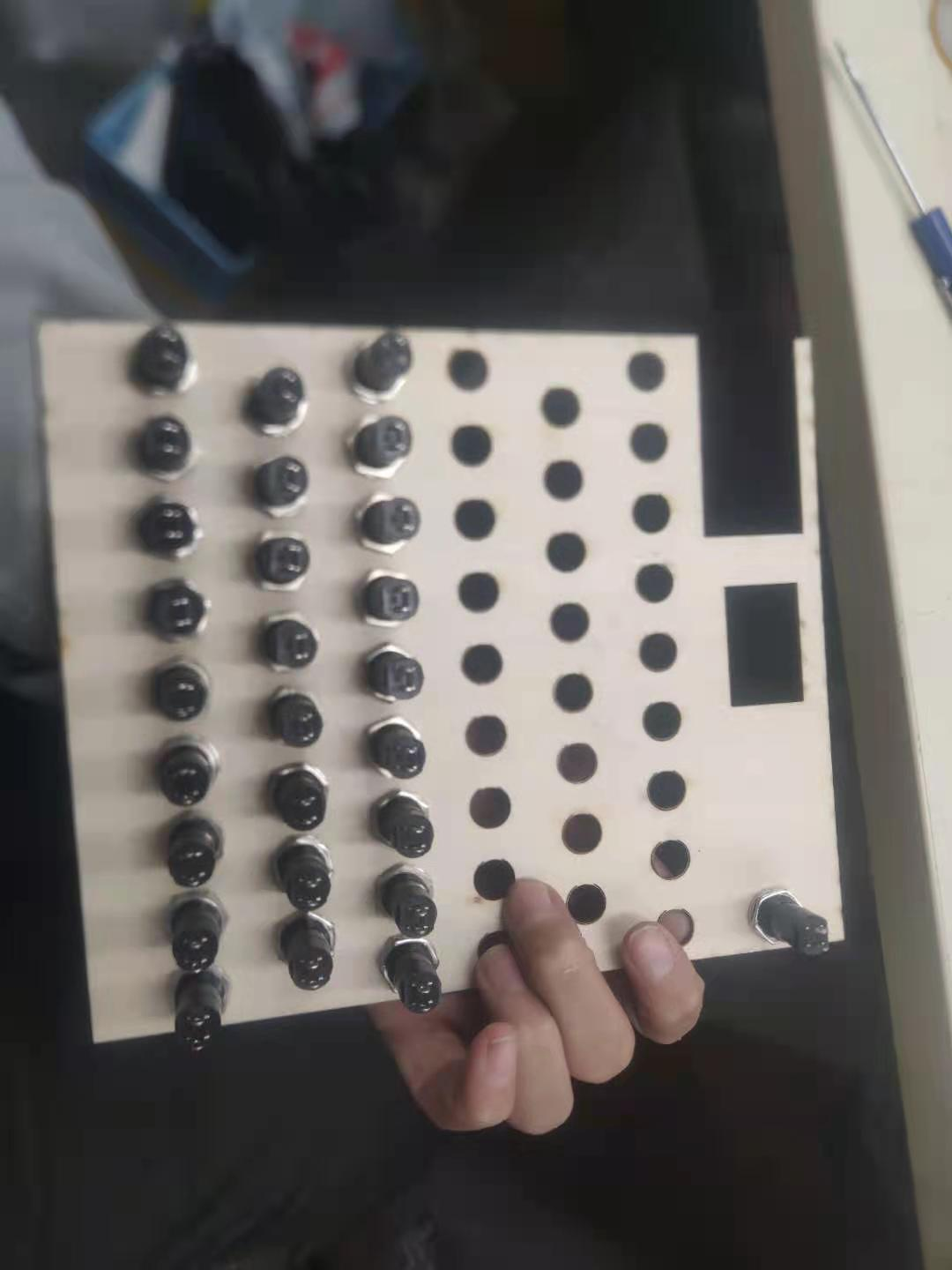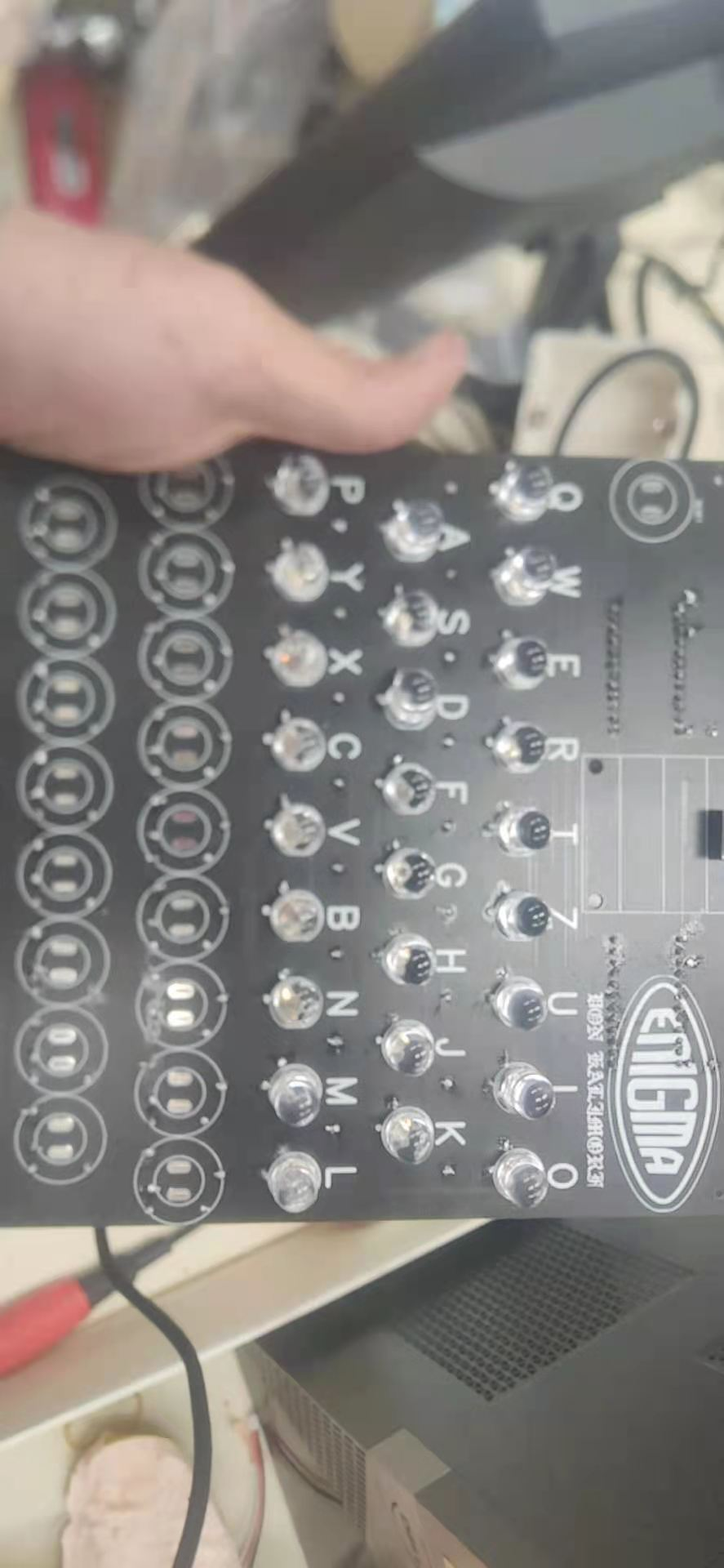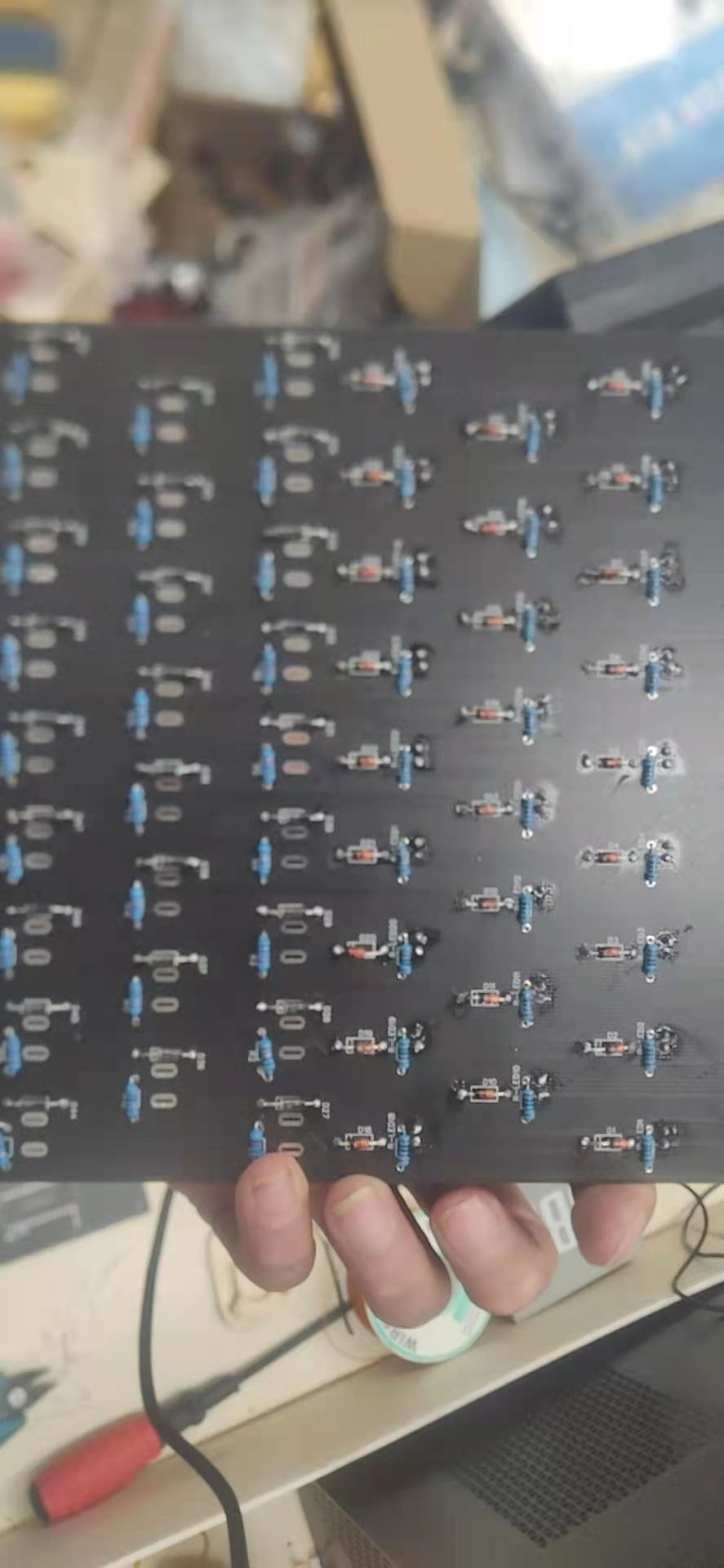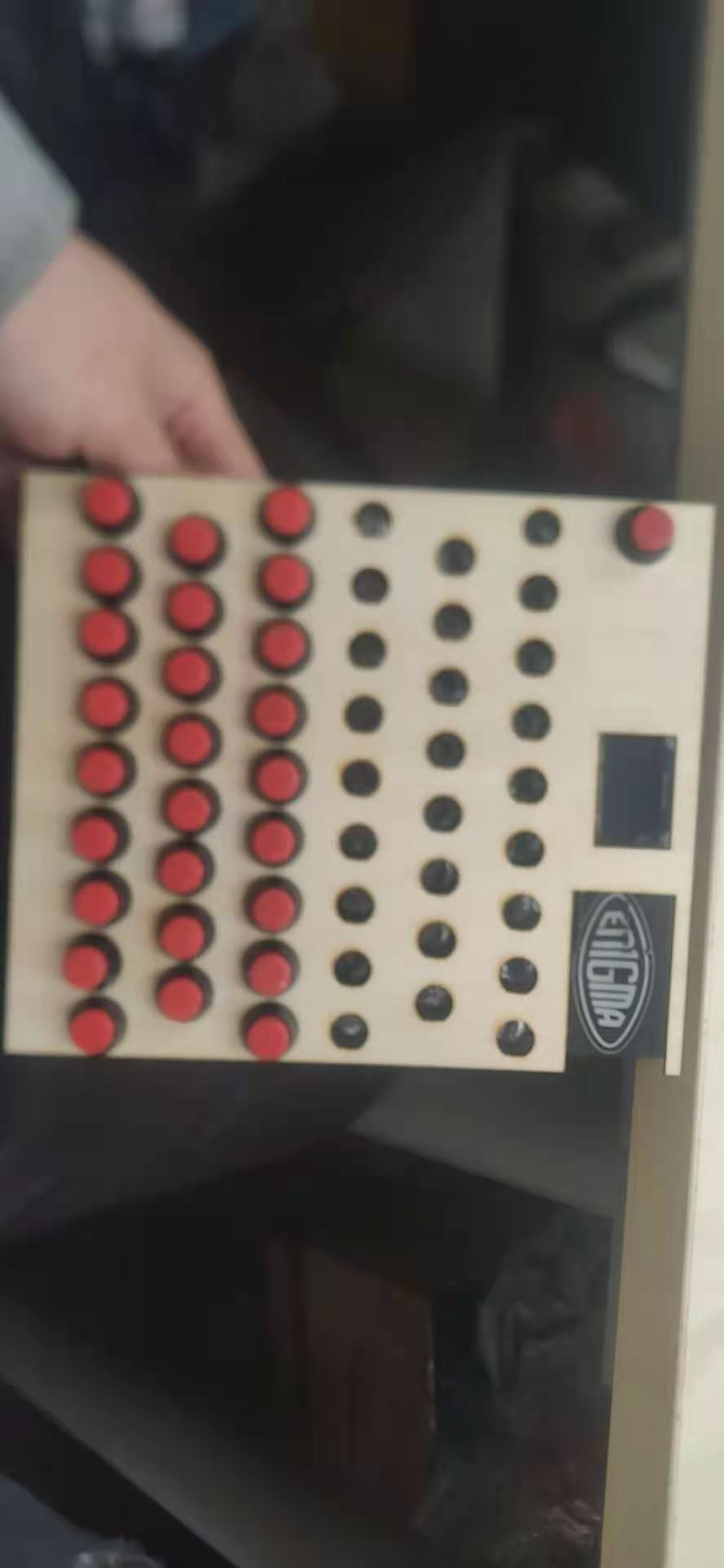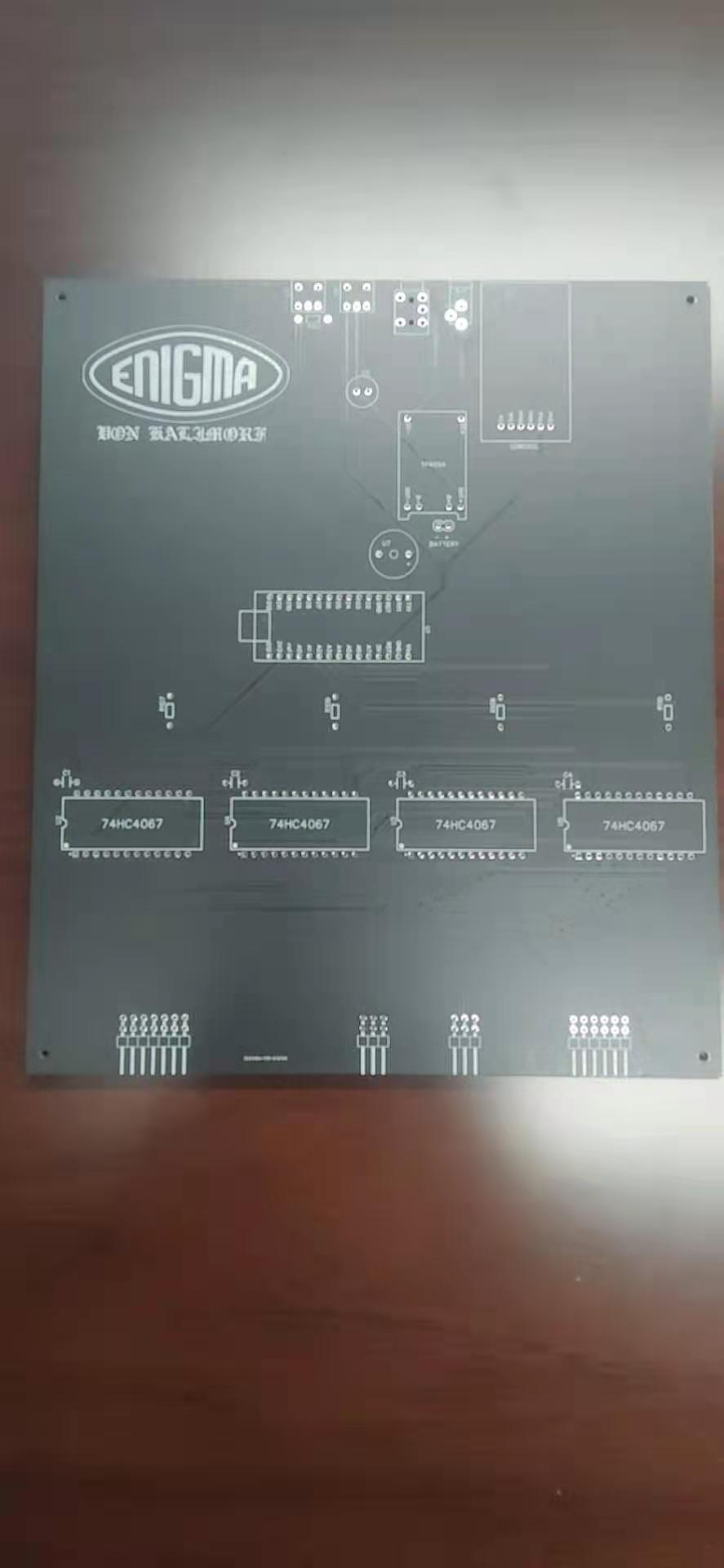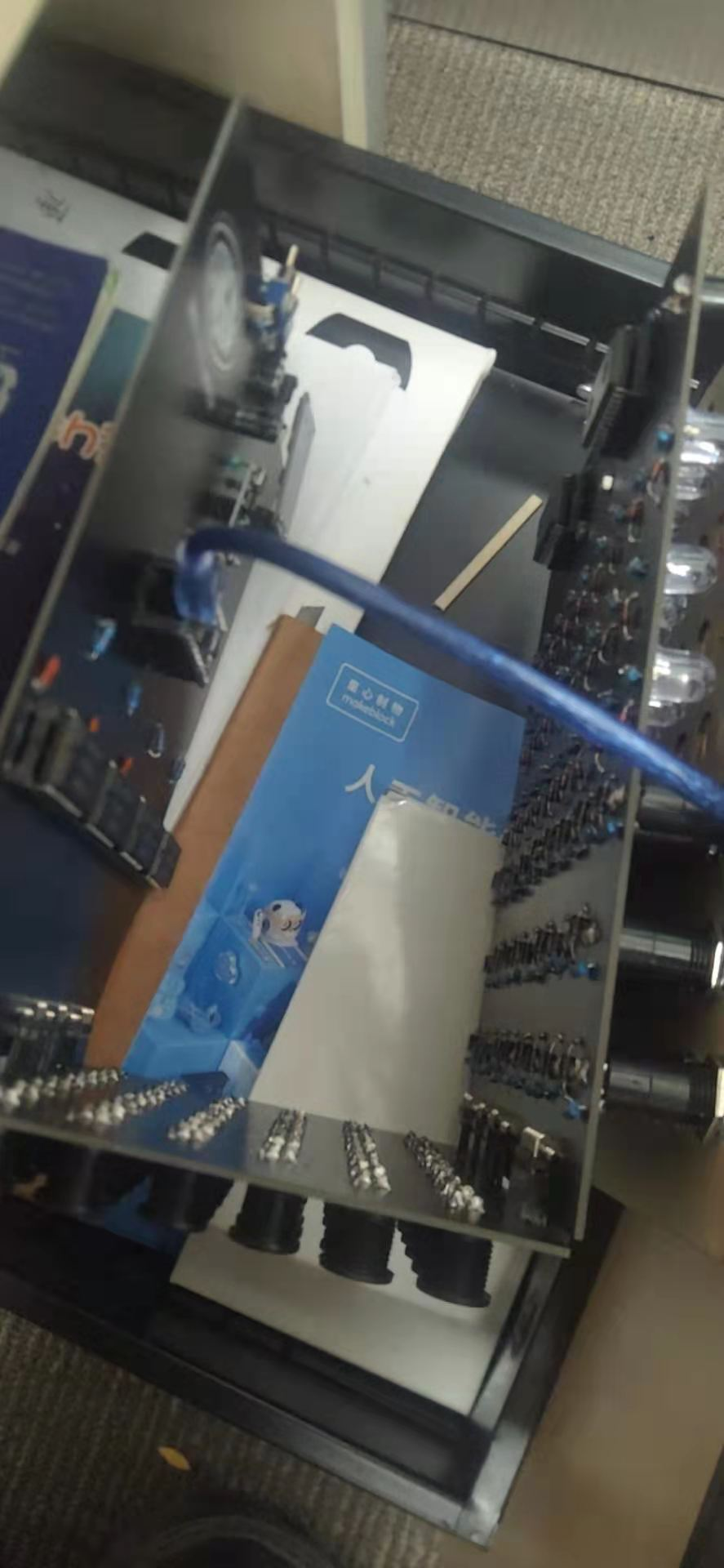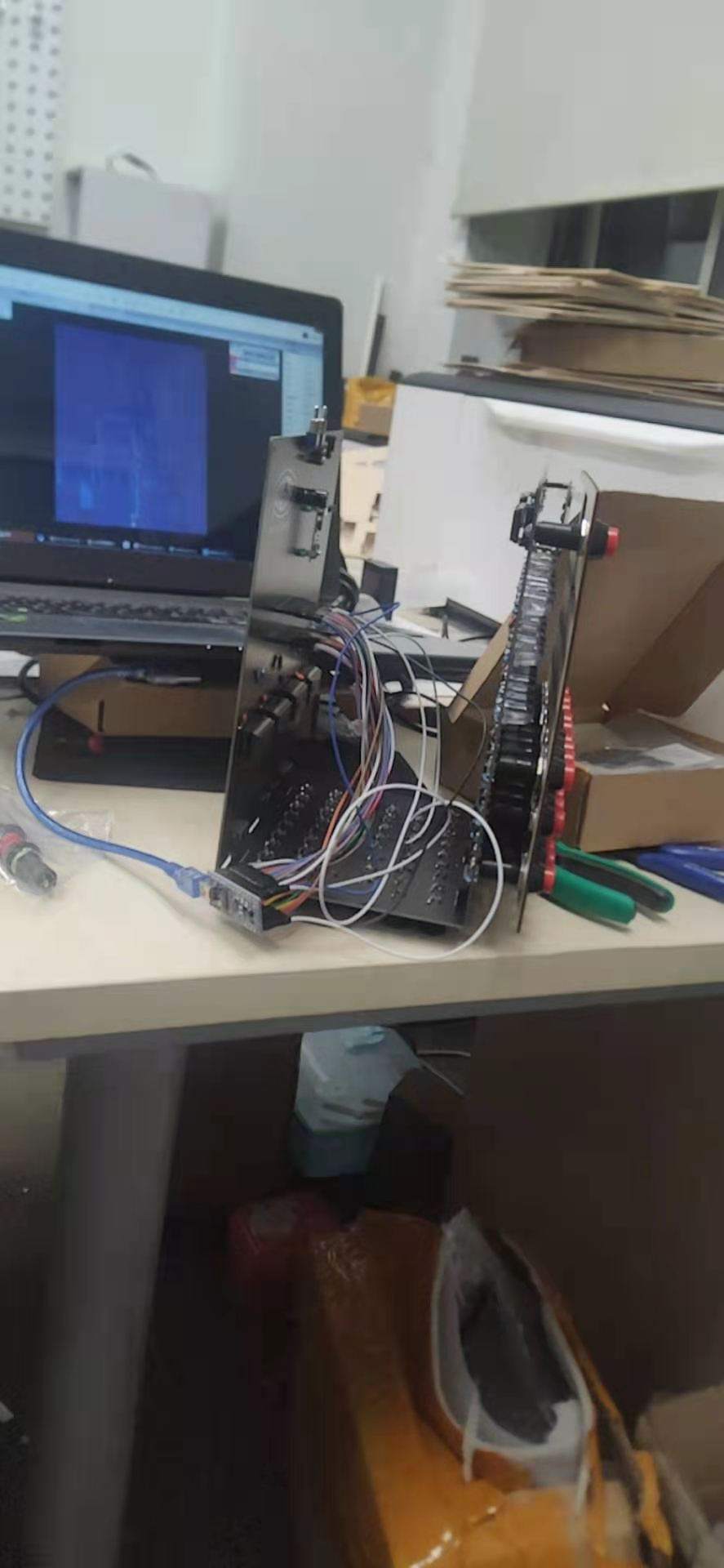```	                    					```#include <EEPROM.h>

char r1 = {10,2,1,16,15,4,9,21,8,22,19,0,23,7,18,5,25,14,12,20,6,17,3,11,24,13};
char r2 = {7,2,6,16,17,9,13,1,23,5,4,14,3,8,20,24,18,10,21,22,12,19,25,11,0,15};
char r3 = {12,4,22,20,25,2,3,17,1,15,0,10,14,5,7,18,9,8,6,11,24,13,16,23,19,21};
char r4 = {2,0,8,19,7,5,22,21,4,17,10,14,1,9,23,20,18,3,16,25,24,12,15,11,6,13};
char r5 = {5,17,3,19,15,11,9,2,1,21,7,0,4,16,22,6,14,25,18,10,12,8,24,13,23,20};
char ref = {25,0,23,15,6,1,17,5,16,18,7,20,21,12,8,9,19,11,4,3,13,10,22,14,24,2};

void setup(){
Serial.begin(9600);
for (int i = 0; i <= 25; i++) {//转子数组五，每组廿六。另有反射器数组一，廿六员。依序排列，录入之。
EEPROM.write(i, r1[i]);
EEPROM.write(i+26, r2[i]);
EEPROM.write(i+52, r3[i]);
EEPROM.write(i+78, r4[i]);
EEPROM.write(i+84, r5[i]);
EEPROM.write(i+110, ref[i]);
}
}

void loop()
{
for (int i = 0; i <= 135; i++) {//反馈之
Serial.print("\t");
}
Serial.println("");
}```
```

```	                    					```#include <EEPROM.h> //动用EEPROM、SD卡、OLED显示屏的库文件
#include <SD.h>
#include "U8glib.h"
U8GLIB_SH1106_128X64 u8g(U8G_I2C_OPT_NO_ACK);

const String Key_mapping[] = {"Q","W","E","R","T","Z","U","I","O","A","S","D","F","G","H","J","K","P","Y","X","C","V","B","N","M","L"}; //键盘字符次序映射表
String final_save; //最终输出储存密文变量

byte Rotor1, Rotor2, Rotor3, Rotor4, Rotor5, Rotor6, Reflictor, Temp; //转子、逆转子、反射器、临时数组
unsigned int counter; //字符计数器
byte Plain = 255; //明文变量
byte Crypto; //密文变量
boolean Key_pressed; //按键状态数组变量
boolean Reflictor_Change = false; //用于初始设定是否有允许反射器和转子转动的设定
boolean Pressed_check = false; //总体按键状态变量
boolean Calculated = false; //加密流程锁变量

void setup() {
// put your setup code here, to run once:
Serial.begin(9600);
pinMode(1, OUTPUT);
pinMode(2, OUTPUT);
pinMode(3, OUTPUT);
pinMode(4, OUTPUT);
pinMode(5, OUTPUT);
pinMode(6, OUTPUT);
pinMode(7, OUTPUT);
pinMode(8, OUTPUT);
pinMode(9, OUTPUT);
pinMode(10, OUTPUT);
pinMode(A0, OUTPUT);
pinMode(A1, OUTPUT);
pinMode(A2, INPUT);
pinMode(A3, INPUT);
digitalWrite(A0, 0);
digitalWrite(A1, 0);

for (byte i = 0; i <= 25; i++) { //此循环用于抄录EEPROM内预装转子编码。
Rotor4[Rotor1[i]] = i; //同时生成几个转子各自的逆运算转子，也用于加密。
Rotor5[Rotor2[i]] = i;
Rotor6[Rotor3[i]] = i;
}

if (!SD.begin(10)) { //报告TF卡是否就位
Serial.println("Card failed");
return;
}
Serial.println("card in");
Serial.end(); //串口终结，其中的TX1口之后用于驱动蜂鸣器播放电码。
}

void Plain_Input_Polling(){ //用轮询监视字母按键按下情况。
for (byte i = 0; i <= 15; i++){ //74HC4067十六通道复用器，以S0~S3收到的高低电平信号来决定某一刻哪个频道导通到公共信号输出端，然后单片机采集这个公共端的数据。为了精简代码节省内存，这里用了根据循环次数计数转化为S0~S3各自地址位是否通断的方式进行。
digitalWrite(2, i % 2);
digitalWrite(3, i / 2 % 2);
digitalWrite(5, i /4 % 2);
digitalWrite(4, i / 8);
if (i <= 9) Key_pressed[i + 16] = (analogRead(A7) >= 650 && analogRead(A7) <= 800); //如果循环次数小于9，则认为可以同时由A7口采集另一片74HC4067的数据，因为两者的S0~S3都是共用单片机的4个管脚的。记录的数据则编入第17~26个字母状态。
}
}

void Key_To_Plain(){ //按键编号到明文生成的子程序
Pressed_check = 0; //按键总体按下情况归零，这个变量用于检测26个按键是否有按下。
for (byte i = 0; i <= 25; i++) {//轮询按键状态数组
Pressed_check = Pressed_check || Key_pressed[i]; //将总体状态和按键状态某个元素进行或运算。只要后者曾经被按下，则总体状态就认定为有按键按下。
if (Pressed_check == 1){ //如果有按键按下
Plain = i; //明文就定义为这个按键编号，反正密码学搞得还是编号数字的学问。
break; //跳出这个循环，反正已经有按键按下去了，怎么检测都是1.
}
}
if (Pressed_check == 0) {//循环完了之后，如果还是检测不到按键。
Plain = 255; //明文回归到初始设定状态
Calculated = 0; //是否进行过加密过程的记录归零，允许一旦接到新的按键就启动加密过程。（避免按着按键不放然后一直自动加密下去。）
}
delay(2); //这一步是消抖消连短暂连击误判的代码。
}

for (byte i = 0; i <= 25; i++) { //首先将转子1转了一格的所有元素顺序存入临时数组内
Temp[i] = Rotor1[(i + 1) % 26]; //其实就是取余运算
}
for (byte i = 0; i <= 25; i++) { //然后将转子1数组赋值为刚刚的临时数组。
Rotor1[i] = Temp[i];
}
for (byte i = 0; i <= 25; i++) { //接着就把它的逆转子排列也生成出来
Rotor4[Rotor1[i]] = i;
}
if (counter % 26 == 0) { //当转子1转了一周后，转子2转一格用来规避密钥重复。同样上面的操作。
for (byte i = 0; i <= 25; i++) {
Temp[i] = Rotor2[(i + 1) % 26];
}
for (byte i = 0; i <= 25; i++) {
Rotor2[i] = Temp[i];
}
for (byte i = 0; i <= 25; i++) {
Rotor5[Rotor2[i]] = i;
}
}
if (counter % 676 == 0) { //当转子2也转了一周后，就轮到转子3转一格。同样上面的操作。
for (byte i = 0; i <= 25; i++) {
Temp[i] = Rotor3[(i + 1) % 26];
}
for (byte i = 0; i <= 25; i++) {
Rotor3[i] = Temp[i];
}
for (byte i = 0; i <= 25; i++) {
Rotor6[Rotor3[i]] = i;
}
}
if (counter % 17576 == 0 && Reflictor_Change == 1) { //如果初始设定连反射器都可以转动的话，那么17576次转子转动后，就进位到反射器也转一格了。
for (byte i = 0; i <= 25; i++) {
Temp[i] = Reflictor[(i + 1) % 26];
}
for (byte i = 0; i <= 25; i++) {
Reflictor[i] = Temp[i];
}
}
}

void Encrypt(){ //这一步是加密顺序流程
Crypto = Rotor1[Plain]; //先将明文经过转子1生成第一阶段的密文
Crypto = Rotor2[Crypto]; //然后依次经过转子2和转子3
Crypto = Rotor3[Crypto];
Crypto = Reflictor[Crypto]; //再经过反射器处理
Crypto = Rotor6[Crypto]; //然后逆回转子3、2、1处理（为了便捷预先生成的逆转子数组用上了）
Crypto = Rotor5[Crypto];
Crypto = Rotor4[Crypto]; //最终得到密文，当然这个密文还是要经过跳线器的，那个步骤不用代码就能在LED上面显示。
}

void Crypto_Indicator(){ //这段子程序决定灯亮哪一盏
digitalWrite(6, (Crypto % 16) % 2); //因为用的还是74HC4067复用器，所以还是可以用取整取余方式进行输出频道控制。
digitalWrite(7, (Crypto % 16) / 2 % 2);
digitalWrite(8, (Crypto % 16) / 4 % 2);
digitalWrite(9, (Crypto % 16) / 8);
digitalWrite(A0, !(Crypto / 16)); //如果字母序号小于16，则这一句就会让A0输出高电平（密文用16除，然后看商是0还是1。用非的原因是需要在小于16的时候输出高电平）
digitalWrite(A1, Crypto / 16); //如果字母序号大于16，则这一句就会让A1输出高电平
}

void Crypto_save(){ //这一段子程序用于将处理密文结果输出到TF卡储存起来
for (byte i = 0; i <= 15; i++){ //因为跳线器的缘故，之前子程序只负责输出灯光，但不知道最后到底是什么灯亮起来。根据电路，额外安装两个74HC4067，用来监测是否有5V电压信号流进LED（经过肖特基二极管，实际到达的电压也就3伏左右。）
digitalWrite(2, i % 2); //和之前控制频道通断机制一样
digitalWrite(3, i / 2 % 2);
digitalWrite(5, i /4 % 2);
digitalWrite(4, i / 8);
Crypto = i; //那就认为最终密文是循环序号，并跳出循环
break;
}
if (i <= 9 && digitalRead(A3) == 1){ //在前十次循环中，如果A3检测到信号，则认为是后十个字母中其中一个是最终密文。
Crypto = i + 16;
break;
}
}
final_save = Key_mapping[Crypto]; //因为电路板实际上的序号不是真正的字母序号，所以需要重新查询回键盘字符映射数组的实际字符元素。
File dataFile = SD.open("OUTPUT.txt", FILE_WRITE); //然后将最终密文字母输出到储存卡里
if (dataFile) {
dataFile.print(final_save);
dataFile.close();
}
}

void Clear(){ //清理按键数据状态数组变量，等待用于下次轮询
for (byte i = 0; i <= 25; i++){
Key_pressed[i] = 0;
}
}

void dot(){ //摩斯电码的短信号
digitalWrite(1, HIGH);
delay(80);
digitalWrite(1, LOW);
delay(80);
}

void dash(){ //摩斯电码的长信号
digitalWrite(1, HIGH);
delay(320);
digitalWrite(1, LOW);
delay(80);
}

void Morse_output(byte code){ //摩斯电码实际输出程序调用，这里已匹配好键盘字符次序映射表
switch (code){
case 0:
dash();dash();dot();dash();
break;
case 1:
dot();dash();dash();
break;
case 2:
dot();
break;
case 3:
dot();dash();dot();
break;
case 4:
dash();
break;
case 5:
dash();dash();dot();dot();
break;
case 6:
dot();dot();dash();dash();
break;
case 7:
dot();dot();
break;
case 8:
dash();dash();dash();
break;
case 9:
dot();dash();
break;
case 10:
dot();dot();dot();
break;
case 11:
dash();dot();dot();
break;
case 12:
dot();dot();dash();dot();
break;
case 13:
dash();dash();dot();
break;
case 14:
dot();dot();dot();dot();
break;
case 15:
dot();dash();dash();dash();
break;
case 16:
dash();dot();dash();
break;
case 17:
dot();dash();dash();dot();
break;
case 18:
dash();dot();dash();dash();
break;
case 19:
dash();dot();dot();dash();
break;
case 20:
dash();dot();dash();dot();
break;
case 21:
dot();dot();dot();dash();
break;
case 22:
dash();dot();dot();dot();
break;
case 23:
dash();dot();
break;
case 24:
dash();dash();
break;
case 25:
dot();dash();dot();dot();
break;
}
}

void loop(){ //主程序
u8g.firstPage(); //OLED屏循环大嵌套
do{
u8g.setFont(u8g_font_unifont); //显示当前转子顺序状态
u8g.setPrintPos(10,40);
u8g.print("                ");
u8g.setPrintPos(10,40);
u8g.print(Key_mapping[Reflictor]);
u8g.setPrintPos(30,40);
u8g.print(Key_mapping[Rotor3]);
u8g.setPrintPos(50,40);
u8g.print(Key_mapping[Rotor2]);
u8g.setPrintPos(70,40);
u8g.print(Key_mapping[Rotor1]);
Plain_Input_Polling(); //进行轮询
Key_To_Plain(); //转化为明文
if (Plain == 255) { //如果没有按键按下，则认为已经进行过加密，并关闭所有LED电压输出
Calculated = 0; //将加密流程锁解除
digitalWrite(A0, LOW);
digitalWrite(A1, LOW);
}
else { //而有按键按下的时候
if (Calculated == 0) { //且加密流程锁已经解除，则进行下列流程
counter++; //字符计数增加1
Encrypt(); //加密
Crypto_Indicator(); //面板显示密文
Crypto_save(); //将密文储存到TF卡里
Morse_output(Crypto); //然后摩斯电码输出
Calculated = 1; //将加密流程锁上锁
}
Clear(); //清理按键状态数组变量
} while(u8g.nextPage());
}```
```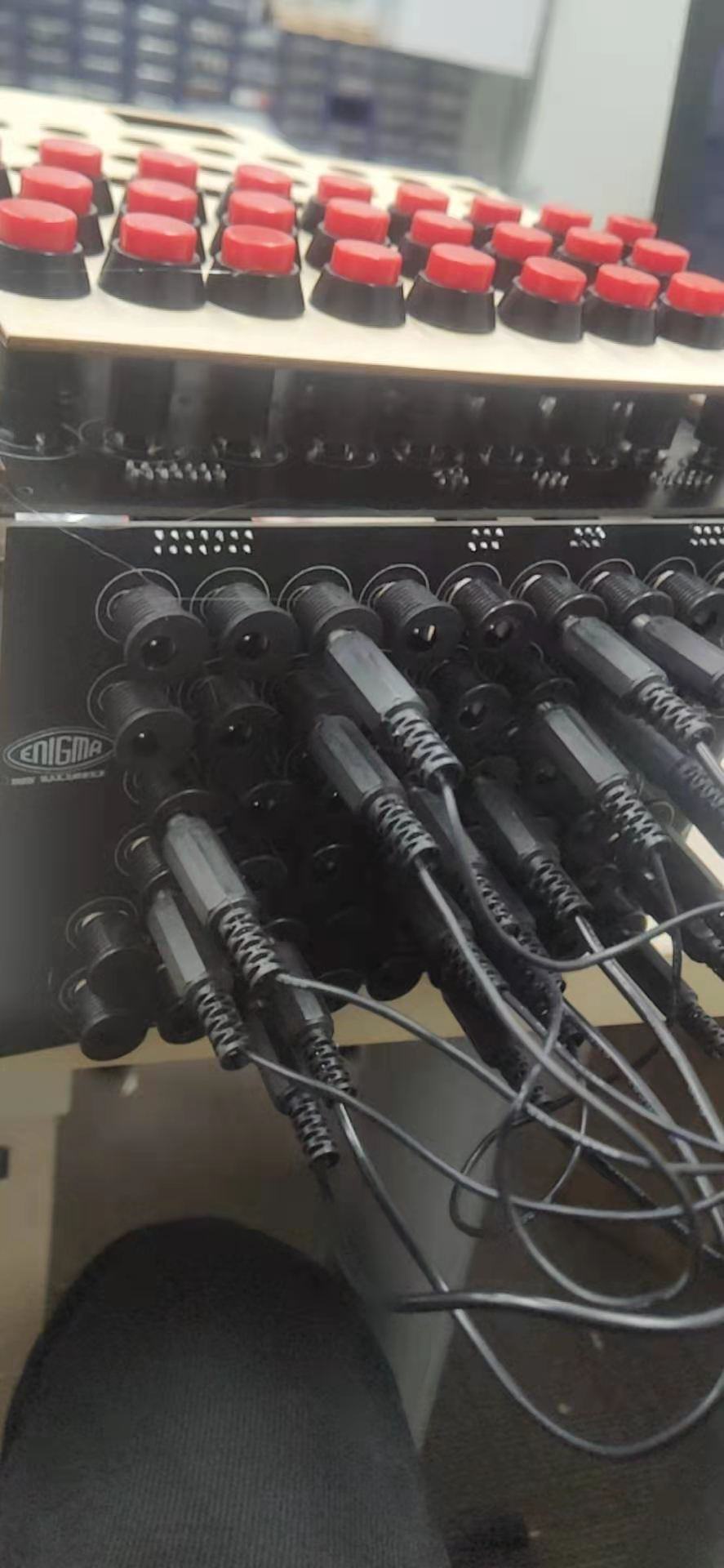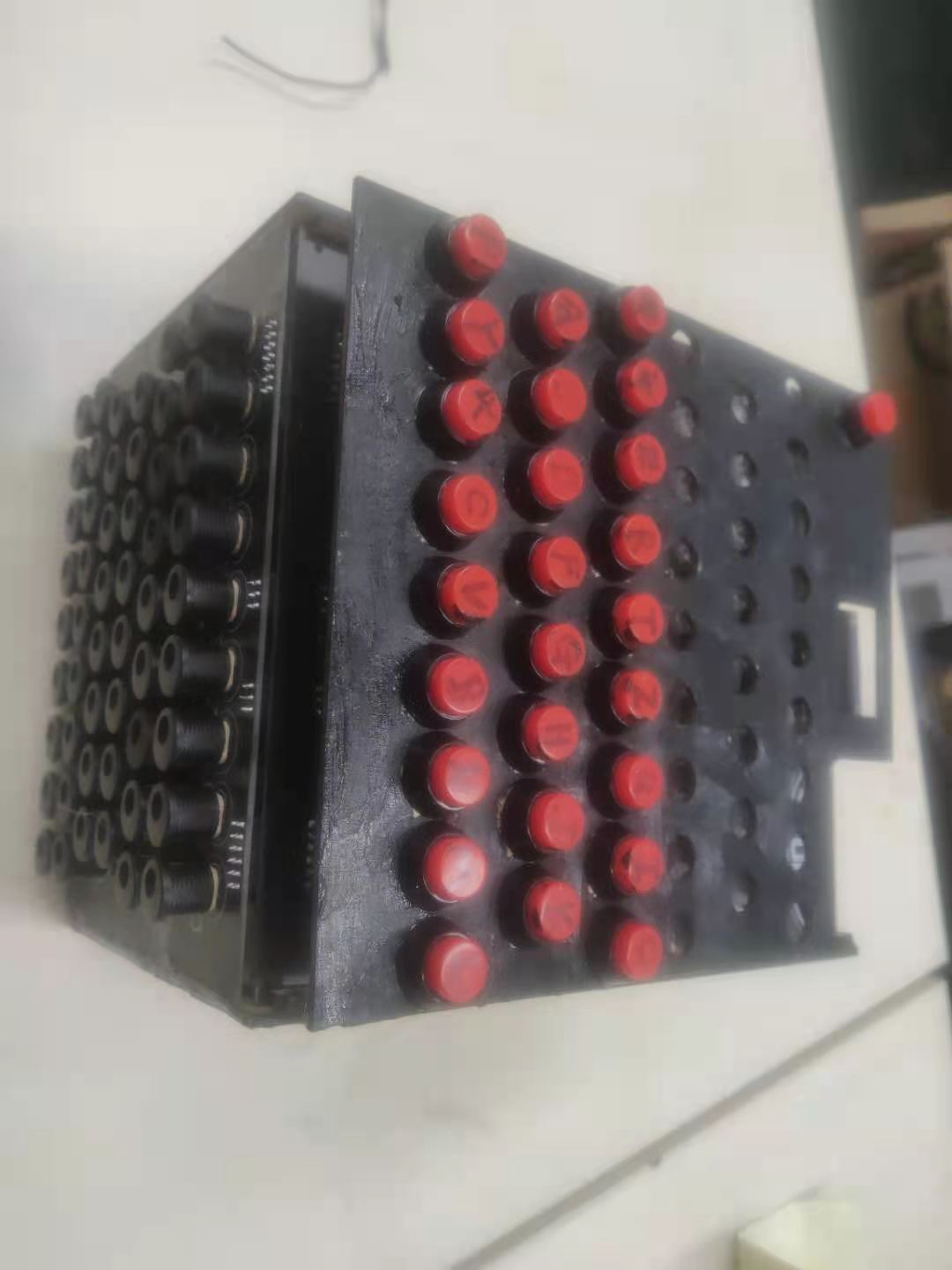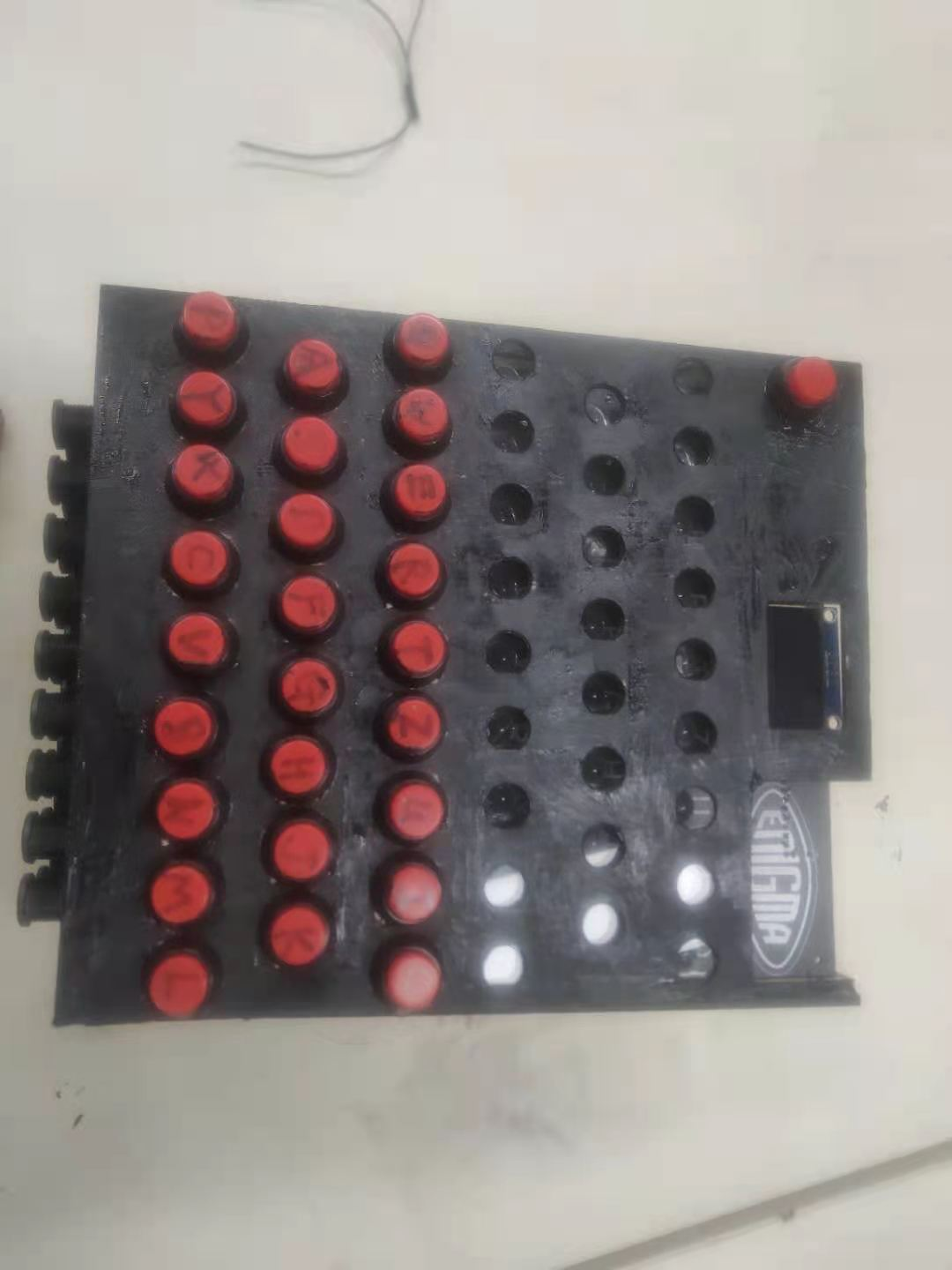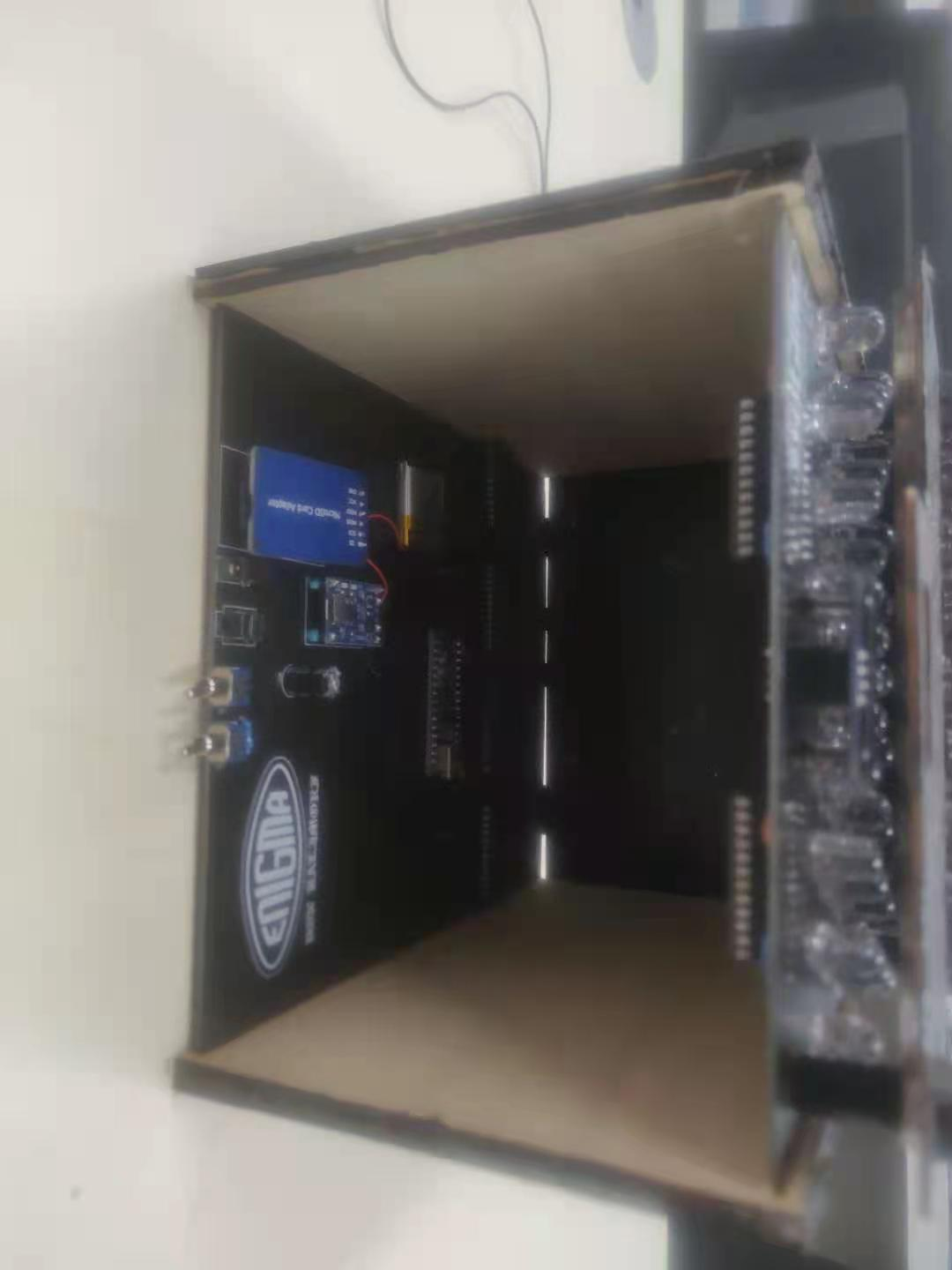Makelog作者原创文章，未经授权禁止转载。
12
1[[c.user_name]] [[c.create_time]]
[[c.parent_comment.count]]
|
[[c.comment_content]]
[[r.user.user_name]] [[r.create_time]]
[[r.comment_content]]

0
10
5
13
4
8
0
13
2
5